Refer to our Texas Go Math Grade 1 Answer Key Pdf to score good marks in the exams. Test yourself by practicing the problems from Texas Go Math Grade 1 Lesson 4.2 Answer Key Model Adding To.

Essential Question
How can you model odding to, or joining to, a group?
Explanation:
With the help of blocks we can add

Explore
Use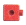to show adding to, or joining.

For The Teacher

• Read the following problem. Have children use connecting cubes to model the problem, and draw to show their work. There are 6 children on the playground. 2 more children join them. How many children are on the playground?
Explanation: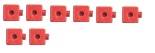6 + 2 = 8
8 children are on the playground

Math Talk
Mathematical Processes
Explanation:

Model and Draw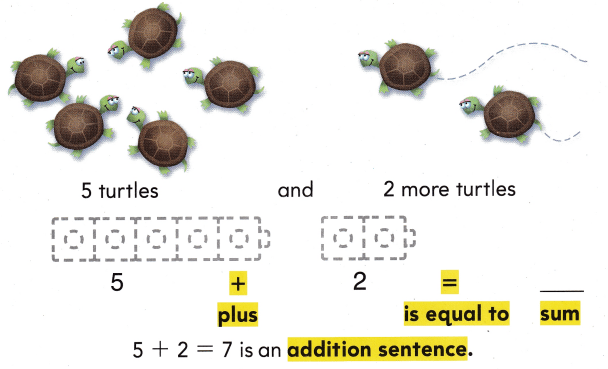Explanation:
5 + 2 = 7
The sum of 5 and 2 is 7

Share and Show

Useto show adding to, or joining. Draw the. Write the sum.

Question 1.
3 cats and 1 more cat

3 + 1 = ___Explanation:
The sum of 3 and 1 is 4
3 + 1 = 4

Question 2.
2 birds and 3 more birds

2 + 3 = __Explanation:
The sum of 2 and 3 is 5
2 + 3 = 5

Question 3.
4 bugs and 4 more bugs

4 + 4 = ___Explanation:
The sum of 4 and 4 is 8
4 + 4 = 8

Question 4.
4 fish and 2 more fish

4 + 2 = ___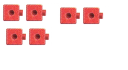Explanation:
The sum of 4 and 2 is 6
4 + 2 = 6

Problem Solving

Use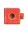to show adding to, or joining. Draw theWrite the sum.

Question 5.
5 bees and 4 more bees

5 + 4 = ___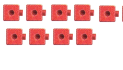Explanation:
The sum of 5 and 4 is 9
5 + 4 = 9

Question 6.
4 dogs and 3 more dogs
4 + 3 = ___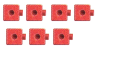Explanation:
The sum of 4 and 3 is 7
4 + 3 = 7

Write or draw to solve.

Question 7.
Molly puts 3 grapes in the bowl. Then she puts 5 more grapes in the bowl. How many grapes are in the bowl?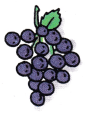____ grapes
Explanation:
3 +5 = 8
8 grapes are in the bowl

Question 8.
H.O.T. Multi-Step Julia has 4 books on the table. She puts 1 more book on the table. Then she puts 2 more BSra books on the table. How many books are on the table?____ books
Explanation:
4 + 1 + 2 = 7
7 books on the table

Question 9.
Chase sees 4 frogs. 2 frogs are green. 2 frogs are brown. Then he sees 1 more brown frog. How many brown frogs does he see?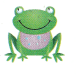___ brown frogs
Explanation:
2 + 1 = 3
3 brown frogs that he see

Question 10.
There are 6 zebras eating grass. Then 3 more zebras join them. How many zebras are eating grass?
(A) 8
(B) 9
(C) 10
Explanation:
6 + 3 = 9
9 zebras are eating grass

Question 11.
Use Tools Which number sentence does the picture show?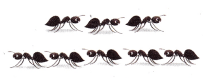(A) 3 + 1 = 4
(B) 3 + 5 = 8
(C) 5 + 5 = 10
Explanation:
3 + 5 = 8
is the sentence that the picture show

Question 12.
Annie picks 5 flowers. Then she picks 2 more. How many flowers does Annie have?
(A) 7
(B) 9
(C) 8Explanation:
5 + 2 = 7
7 flowers that Annie have

Question 13.
Texas Test Prep Use to show adding to, or joining. What is the sum of 4 and 3?
(A) 1
(B) 7
(C) 6
Explanation:
4 + 3 = 7
the sum of 4 and 3 is 7

Take Home Activity

• Put 3 pennies in one group and 2 pennies in another group. Have your child write an addition sentence to tell about the pennies. Repeat for other combinations of pennies with sums up to 10.

### Texas Go Math Grade 1 Lesson 4.2 Homework and Practice Answer Key

Use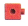to show adding to. Draw the. Write the sum.

Question 1.
1 monkey and 5 more monkeys

1 + 5 = ___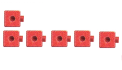Explanation:
The sum of 1 and 5 is 6
1 + 5 = 6

Question 2.
3 camels and 6 more camels

3 + 6 = ___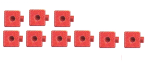Explanation:
The sum of 3 and 6 is 9
3 + 6 = 9

Problem Solving

Write or draw to solve.

Question 3.
Kevin throws the ball to his dog 4 times. Then he throws the ball 4 more times. How many times does he throw the ball?
___ times
Explanation:
4 + 4 = 8
8 times that  he throw the ball

Question 4.
Multi-Step Emily buys 5 shirts. 2 are pink. 3 are blue. Then she buys 2 more shirts. They are red. How many shirts does she buy?
___ shirts
Explanation:
5 + 2 = 7 shirts she buy

Lesson Check

Texas Test Prep

Question 5.
There are 8 children reading. Then I more child joins them. How many children are reading?
(A) 7
(B) 10
(C) 9
Explanation:
8 + 1 = 9

Question 6.
Which number sentence does the picture show?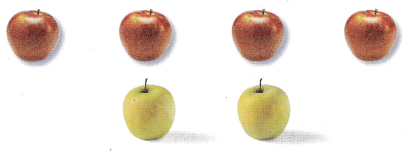(A) 4 + 3 = 7
(B) 4 + 2 = 6
(C) 4 + 5 = 9
Explanation:
4 + 2 = 6
Is the number sentence that the picture show

Question 7.
What is the sum of 3 and 6?
(A) 3
(B) 9
(C) 8
Explanation:
the sum of 3 and 6 is 9

Question 8.
Multi-Step Evan has 5 video games. His brother gives him 2 games. Then he buys 1 more game. How many games does Evan have?
(A) 8
(B) 2
(C) 7